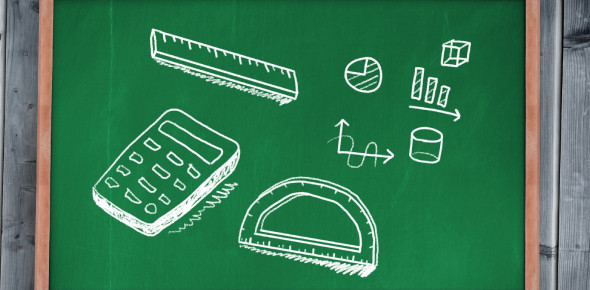# Quiz: Solve The Basic Geometry Questions

12 Questions | Attempts: 469
ShareSettingsAre you ready to solve the basic geometry questions? Then take this quiz. Geometry is a huge part of mathematics. The quiz here has some basic questions to test your knowledge of the basics of geometry. The quiz will also make your base even stronger on the same. Wish you the best of luck with these questions. Let's go for it and learn something more as well as have fun!

• 1.
Which formula is used to find the area of a parallelogram?
• A.
• B.
• C.
• D.
• 2.
Mrs. Porter is painting purple circles on the sidewalk with a diameter of 14 inches.  What is the area of the circles?
• A.
• B.
• C.
• D.
• 3.
James has a square rug that measures 12.6 feet on each side.  Find the area of the rug.
• A.
• B.
• C.
• D.
• 4.
Which formula is used to find the area of a circle?
• A.
• B.
• C.
• D.
• 5.
Which formula is used to find the area of a parallelogram?
• A.
• B.
• C.
• D.
• 6.
Which formula is used to find the area of a triangle?
• A.
• B.
• C.
• D.
• 7.
Which formula is used to find the area of a trapezoid?
• A.
• B.
• C.
• D.
• 8.
Which formula is used to find the area of a rectangle?
• A.
• B.
• C.
• D.
• 9.
Find the area of a rectangle with a base of 2.5 cm and a height of 8.1 cm.
• A.
• B.
• C.
• D.
• 10.
• A.
• B.
• C.
• D.
• 11.
The students had a relay race on field day. The race was arranged in a triangular formation. It had a base of 25 yards and a height of 50 yards. What was the area of the race field?
• A.
• B.
• C.
• D.
• 12.
The roof of a doghouse is shaped like a parallelogram.  The roof has a base of 4.5 feet and a height of 5.2 feet.  What is the area of the roof of the doghouse?
• A.
• B.
• C.
• D.

## Related TopicsBack to top
×

Wait!
Here's an interesting quiz for you.